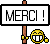[Livre] Step-by-Step Basic Statistics Using SAS: Student Guide
Bonjour,Description :

Larry Hatcher's Step-by-Step Basic Statistics Using SAS: Student Guide first introduces you to SAS software, then leads you through a variety of elementary statistical analyses that are commonly used in the social and behavioral sciences. With SAS, you can focus more on conceptual issues in statistical analysis and less on the mechanics of performing mathematical operations by hand. Main topics include how to use the SAS windowing environment to write and submit SAS programs; how to create SAS data sets and modify the variables that they contain; basic descriptive statistics (measures of central tendency and variability, frequency tables, and graphs); correlation and regression; t tests (single-sample, independent and paired samples); analysis of variance (ANOVA), both one-way and factorial; and the chi-square test of independence. Even if you are new to statistics and computers, you soon will be recording research data, writing SAS programs, and interpreting the results. Reinforce what you've learned by completing the exercises in the companion text, Step-by-Step Basic Statistics Using SAS: Exercises. Larry Hatcher's Step-by-Step Basic Statistics Using SAS: Student Guide first introduces you to SAS software, then leads you through a variety of elementary statistical analyses that are commonly used in the social and behavioral sciences. With SAS, you can focus more on conceptual issues in statistical analysis and less on the mechanics of performing mathematical operations by hand. Main topics include how to use the SAS windowing environment to write and submit SAS programs; how to create SAS data sets and modify the variables that they contain; basic descriptive statistics (measures of central tendency and variability, frequency tables, and graphs); correlation and regression; t tests (single-sample, independent and paired samples); analysis of variance (ANOVA), both one-way and factorial; and the chi-square test of independence. Even if you are new to statistics and computers, you soon will be recording research data, writing SAS programs, and interpreting the results. Reinforce what you've learned by completing the exercises in the companion text, Step-by-Step Basic Statistics Using SAS: Exercises.

• Avez-vous lu ce livre ou pensez-vous le lire ?
• Souhaitez-vous ajouter une critique de ce livre sur la page Livres de la rubrique ?
• Avez-vous un commentaire à faire ?